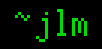## 25-Jan-2006

### Combinatorics: Counting with replacement

Filed under: math — jlm @ 05:14

Everyone knows the number of ways you can choose k items out of n without replacement is nCk = n!/k!(n-k)!. Often forgotten is that the number of ways to choose k items from n with replacement is n+k-1Ck. I think this is because a) it doesn’t come up as much, and b) there’s a clear intuitition behind the first formula but not so the second. So, why is the value n+k-1Ck? Proving it with induction is a simple matter, but doesn’t shed much light.

Let’s look at it differently… We can number our items 1, 2, …, n and order the k chosen items accordingly, making a non-decreasing sequence. So the problem becomes counting the non-decreasing sequences a1a2 ≤ … ≤ ak. Turn each sequence into a bar graph, so that for n=7, k=4 the sequence [3,5,5,6] becomesWe can make the graph into a path from (0,1) to (k,n)This path has n-1+k steps, n-1 vertical and k horizontal. Choosing one such path is choosing the k horizontal steps from the n+k-1 total, hence n+k-1Ck.

Another way to think about it is to consider the items chosen to be bins to fill with balls: Choosing at item becomes putting a ball in the bin, and the number of balls in a bin represents how many times that item was chosen. So the problem becomes count the number of ways you can put k identical balls in n bins. Start with bin 1. At each step you can put a ball into the current bin, or move to the next bin. You’ll place balls in k of the steps, and move bins in n-1 of them, so it’s a matter of choosing the k steps out of the k+n-1 total in which to place balls.